# 《Python程序设计与算法基础教程（第二版）》江红 余青松 全部章节的课后习题，上机实践，课后答案，案例研究

（还在更新中…） 这篇博客花费了我的大量时间和精力，从创作到维护；若认可本篇博客，希望给一个点赞、收藏

1. 几个例题： 假装自己从零开始学，将一些有代表性、有意思的例题抽取出来
2. 部分复习题： 遇到有意思的复习题，我会拿出来，并且进行分析
3. 上机实践： 全部上机实践题的解题思路

# 第一章 Python概述

## 几个例题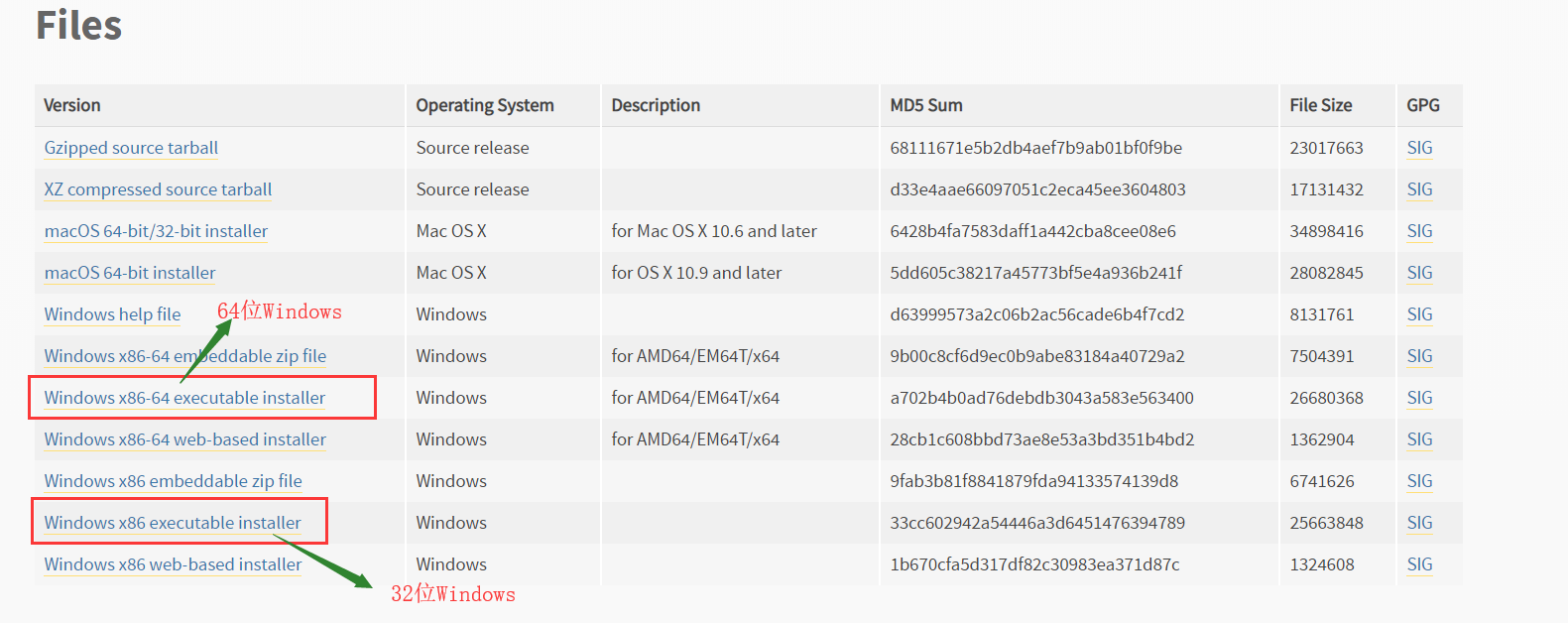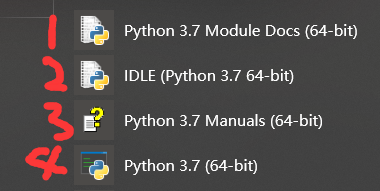1. Python 3.7 Module Docs（64-bit）
点击之后，会出现一个网页（将我下载的Python3.7.4文件夹中包含的模块都列了出来,页面不止这么点，还可以往下拉）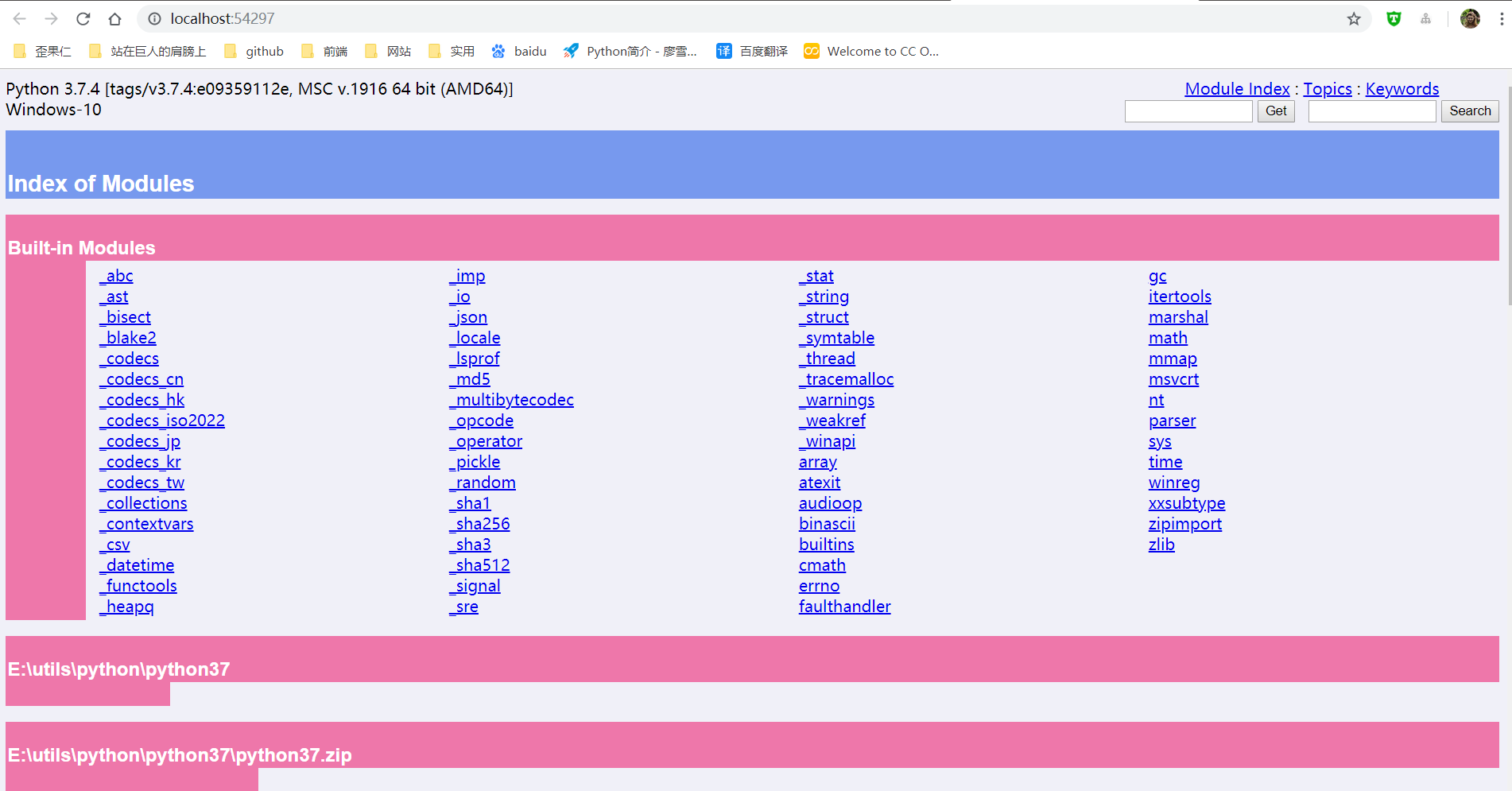2. IDLE（Python 3.7 64-bit）
一个Python编辑器，Python内置的集成开发工具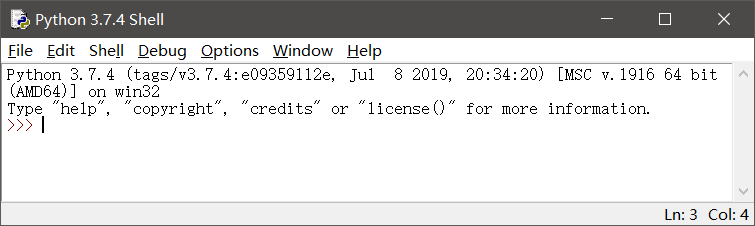3. Python 3.7 Manuals（64-bit）
Python 3.7 开发手册

4. Python 3.7（64-bit）
控制台中运行Python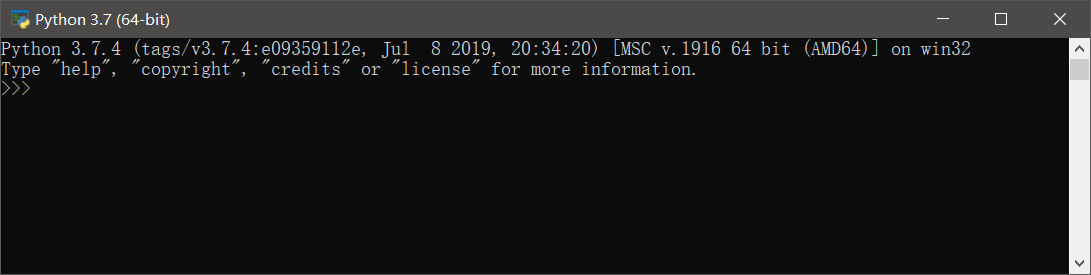1. pip用于安装和管理Python扩展包
2. setuptools用于发布Python包
python -m pip install -U pip setuptools


Python扩展模块NumPy提供了数组和矩阵处理，以及傅立叶变换等高效的数值处理功能

 python -m pip install NumPy


Matplotlib是Python最著名的绘图库之一，提供了一整套和MATLAB相似的命令API，既适合交互式地进行制图，也可以作为绘图控件方便地嵌入到GUI应用程序中

python -m pip install Matplotlib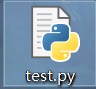1. 右键test.py---->Edit With IDLE---->Edit With IDLE 3.7（64-bit）
2. 打开IDLE，然后File---->Open（或者ctrl+O）选择.py文件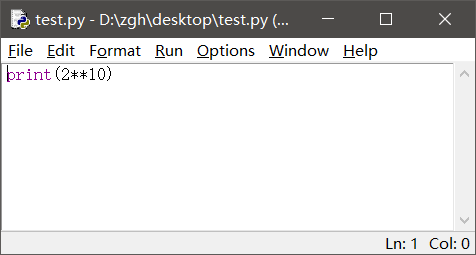Run---->Run Module（或者F5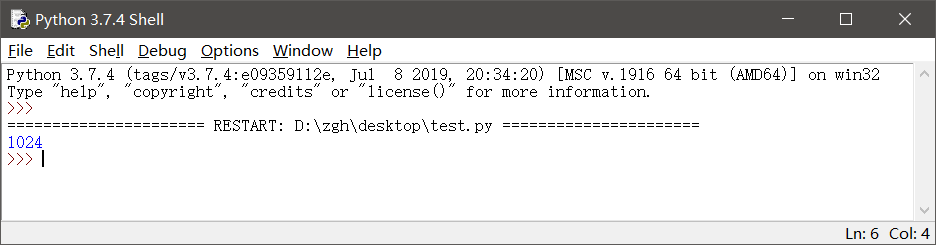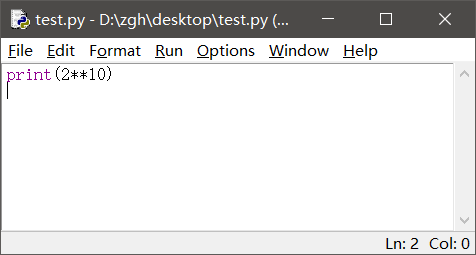hello.py文件在桌面

import random

print("hello,Python")
print("你今天的随机数字是：",random.choice(range(10)))#输出在0-9之间随机选择的整数
input()

1. 在桌面打开PowerShell（还有两种输入方式：python hello.py或者.\hello.py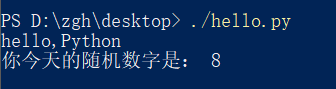2. 或者在桌面打开cmd, 就输入hello.py或者python hello.py

hello_argv.py文件在桌面

import sys

print("Hello,",sys.argv)
#这样写也行：
#print("Hello,"+sys.argv)

1. 在桌面打开PowerShell（还有两种输入方式：python hello_argv.py 任意输入或者./hello_argv.py 任意输入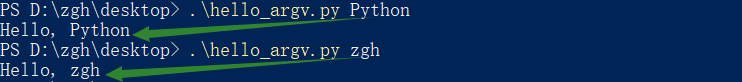2. 或者在桌面打开cmd，就输入hello_argv.py 任意输入或者python hello_argv.py 任意输入

# 第二章 Python语言基础

## 选择题：1、3、7、8

1. 在Python中，以下标识符合法的是

A. _B. 3CC. it’sB. str

1. 标识符的第一个字符必须是字母，下划线（_）；其后的字符可以是字母、下划线或数字。
2. 一些特殊的名称，作为python语言的保留关键字，不能作为标识符
3. 以双下划线开始和结束的名称通常具有特殊的含义。例如__init__为类的构造函数，一般应避免使用

B：以数字开头，错误
C：使用了'，不是字母、下划线或数字
D：str是保留关键字

3. 在下列Python语句中非法的是

A. x = y =1B. x = (y =1)C. x,y = y,xB. x=1;y=1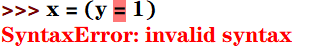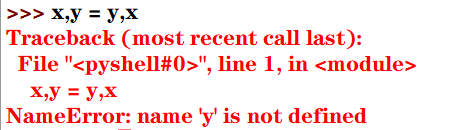7. 为了给整型变量x，y，z赋初值10，下面Python赋值语句正确的是

A. xyz=10B. x=10 y=10 z=10C. x=y=z=10B. x=10，y=10，z=10

1. 分号;用于在一行书写多个语句
2. python支持链式赋值

A：赋值对象是xyz
B：分号;用于在一行书写多个语句，而不是' '（即空格）
D：分号;用于在一行书写多个语句，而不是,

8. 为了给整型变量x，y，z赋初值5，下面Python赋值语句正确的是

A. x=5;y=5;z=5B. xyz=5C. x,y,z=10B. x=10，y=10，z=10

Pytho能支持序列解包赋值，但是变量的个数必须与序列的元素个数一致，否则会报错

B：赋值对象是xyz
C：序列解包赋值，变量的个数必须与序列的元素个数一致，否则会报错
D：分号;用于在一行书写多个语句，而不是,

## 思考题：9

9.下列Python语句的输出结果是

def f():pass
print(type(f()))


NoneType数据类型包含唯一值None，主要用于表示空值，如没有返回值的函数的结果

## 上机实践：2~6

2. 编写程序，输入本金、年利率和年数，计算复利（结果保留两位小数）

money = int(input("请输入本金："))
rate = float(input("请输入年利率："))
years = int(input("请输入年数："))
amount = money*((1+rate/100)**years)
print(str.format("本金利率和为：{0:2.2f}",amount))


请输入本金：1000



3. 编写程序，输入球的半径，计算球的表面积和体积（结果保留两位小数）

import math
r = float(input("请输入球的半径："))
area = 4 * math.pi * r**2
volume = 4/3*math.pi*r**3
print(str.format("球的表面积为：{0:2.2f}，体积为：{1:2.2f}",area,volume))


请输入球的半径：666



4. 编写程序，声明函数getValue(b,r,n)，根据本金b，年利率r和年数n计算最终收益v

money = int(input("请输入本金："))
rate = float(input("请输入年利率(<1)："))
years = int(input("请输入年数："))

def getValue(b,r,n):
return b*(1+r)**n

print(str.format("本金利率和为：{0:2.2f}",getValue(money,rate,years)))


请输入本金：10000



5. 编写程序，求解一元二次方程x2-10x+16=0

from math import sqrt
x = (10+sqrt(10*10-4*16))/2
y = (10-sqrt(10*10-4*16))/2
print(str.format("x*x-10*x+16=0的解为：{0:2.2f}，{1:2.2f}",x,y))


x*x-10*x+16=0的解为：8.00，2.00


6. 编写程序，提示输入姓名和出生年份，输出姓名和年龄

import datetime
sName = str(input("请输入您的姓名："))
birthday = int(input("请输入您的出生年份："))
age = datetime.date.today().year - birthday
print("您好！{0}。您{1}岁。".format(sName,age))


请输入您的姓名：zgh



## 案例研究：使用Pillow库处理图像文件

https://blog.csdn.net/Zhangguohao666/article/details/102060722

# 第三章 程序流程控制

## 几个例题

y = int(input("请输入要判断的年份："))
if((y % 4 == 0 and y % 100 != 0) or y % 400 == 0):
print("是闰年")
else:
print("不是闰年")


from calendar import isleap

y = int(input("请输入要判断的年份："))
if(isleap(y)):print("闰年")
else:print("不是闰年")


for i in range(1,10):
s = ""
for j in range(1,10):
s += str.format("%d * %d = %02d  " %(i, j, i*j))
print(s)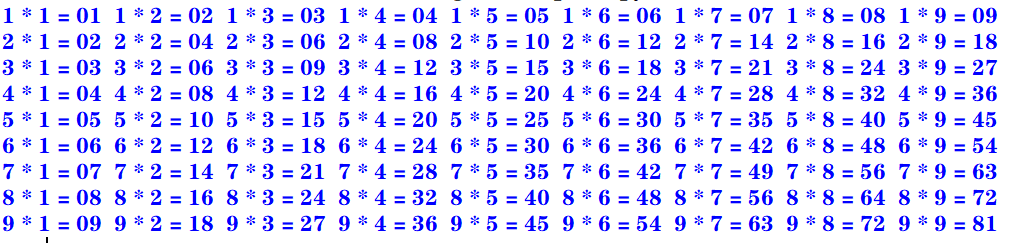for i in range(1,10):
s = ""
for j in range(1,i+1):
s += str.format("%d * %d = %02d  " %(i, j, i*j))
print(s)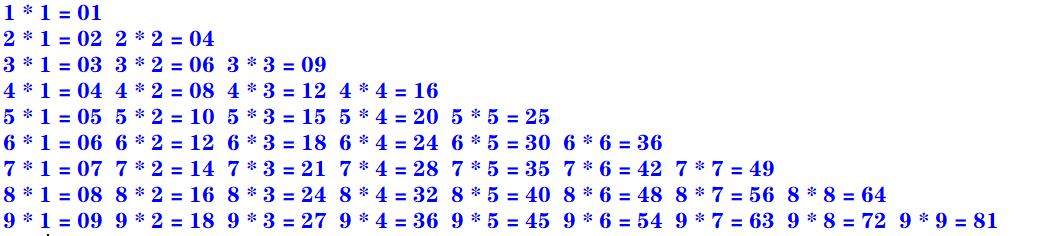for i in range(1,10):
s = ""
for k in range(1,i):
s += "                   "
for j in range(i,10):
s += str.format("%d * %d = %02d  " %(i, j, i*j))
print(s)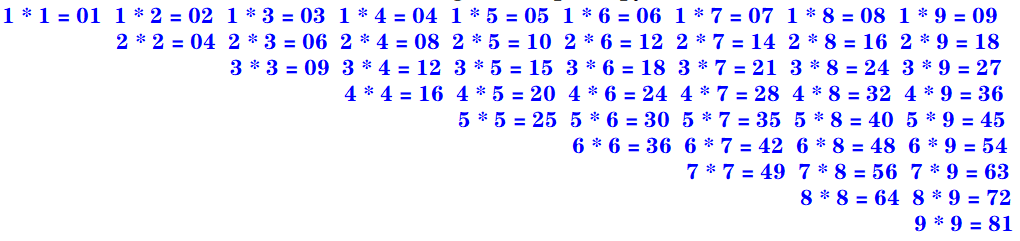Python语言中的for循环直接迭代对象集合中的元素，如果需要在循环中使用索引下标访问集合元素，则可以使用内置的enumerate()函数

enumerate()函数用于将一个可遍历的数据对象（例如列表、元组或字符串）组合为一个索引序列，并返回一个可迭代对象，故在for循环当中可直接迭代下标和元素

seasons = ["Spring","Summer","Autumn","Winter"]
for i,s in enumerate(seasons,start=1):    #start默认从0开始
print("第{0}个季节：{1}".format(i,s))


第1个季节：Spring



zip()函数将多个可迭代对象中对应的元素打包成一个个元组，然后返回一个可迭代对象。如果元素的个数不一致，则返回列表的长度与最短的对象相同。

evens = [0,2,4,6,8]
odds = [1,3,5,7,9]
for e,o in zip(evens,odds):
print("{0} * {1} = {2}".format(e,o,e*o))


0 * 1 = 0
2 * 3 = 6
4 * 5 = 20
6 * 7 = 42
8 * 9 = 72


map(func,seq1[,seq2,...])

• func作用于seq中的每一个元素，并将所有的调用结果作为可迭代对象返回。
• 如果func为None，该函数的作用等同于zip()函数

>>> list(map(abs, [-1, 0, 7, -8]))
[1, 0, 7, 8]


>>> list(map(pow, range(5), range(5)))
[1, 1, 4, 27, 256]


>>> list(map(ord, 'zgh'))
[122, 103, 104]


>>> list(map(lambda x, y: x+y, 'zgh', '666'))
['z6', 'g6', 'h6']


## 选择题：1、2、3

1. 下面的Python循环体的执行次数与其他不同的是

A.

i = 0
while(i <= 10):
print(i)
i = i + 1


B.

i = 10
while(i > 0):
print(i)
i = i - 1


C.

for i in range(10):
print(i)


D.

for i in range(10,0,-1):
print(i)


A：[0,10] 执行11次
B：[10,1] 执行10次
C：[0,9) 执行10次
D：[10,0) 执行10次

2. 执行下列Python语句将产生的结果是

x = 2; y = 2.0
if(x == y): print("Equal")
else: print("Not Equal")

A. EqualB. Not EqualC. 编译错误D. 运行时错误

Python中的自动类型转换：

1. 自动类型转换注意针对Number数据类型来说的
2. 当2个不同类型的数据进行运算的时候,默认向更高精度转换
3. 数据类型精度从低到高:bool int float complex
4. 关于bool类型的两个值：True 转化成整型是1；False 转化成整型是0

int类型的2转化为float类型的2.0

3. 执行下列Python语句将产生的结果是

i= 1
if(i): print(True)
else: print(False)

A. 输出1B. 输出TrueC. 输出FalseD. 编译错误

## 填空题：6

6. 要使语句for i in range(_,-4,-2)循环执行15次，则循环变量i的初值应当为>>> a = 0
>>> for i in range(26, -4, -2): a+=1

>>> print(a)
15

>>> a = 0
>>> for i in range(25, -4, -2): a+=1

>>> print(a)
15


• 如果步长为 -1或者1，那么答案只有一个
• 如果步长为 -2或者2，那么答案有两个
• 如果步长为 -3或者3，那么答案有三个

• 如果步长为2，还要计算 (x ± 1) - z × (result-1) 的值，然后再经过琐碎的判断即可
• 如果步长为3，还要计算 (x ± 2) - z × (result-1) 的值，…

## 思考题：3~6

3. 阅读下面的Python程序，请问程序的功能是什么？

from math import sqrt

n = 0
for m in range(101,201,2):
k = int(sqrt(m))
for i in range(2, k+2):
if m % i == 0:break
if i == k + 1:
if n % 10 == 0:print()
print('%d' % m,end = " ")
n += 1


101 103 107 109 113 127 131 137 139 149
151 157 163 167 173 179 181 191 193 197
199


4. 阅读下面的Python程序，请问输出的结果使什么？

n = int(input("请输入图形的行数："))
for i in range(0, n):
for j in range(0, 10 - i):print(" ", end=" ")
for k in range(0, 2 * i + 1):print(" * ", end=" ")
print("\n")


请输入图形的行数：4
*

*   *   *

*   *   *   *   *

*   *   *   *   *   *   *


5. 阅读下面的Python程序，请问输出的结果使什么？程序的功能是什么？

for i in range(100,1000):
n1 = i // 100
n2 = i // 10 % 10
n3 = i % 10
if(pow(n1, 3) + pow(n2, 3) + pow(n3, 3) == i):print(i, end=" ")


153 370 371 407


6. 阅读下面的Python程序，请问输出的结果使什么？程序的功能是什么？

for n in range(1,1001):
total = 0; factors = []
for i in range(1, n):
if(n % i == 0):
factors.append(i)
total += i
if(total == n):print("{0} : {1}".format(n, factors))


6 : [1, 2, 3]
28 : [1, 2, 4, 7, 14]
496 : [1, 2, 4, 8, 16, 31, 62, 124, 248]


## 上机实践：2~14

2. 编写程序，计算1=2+3+…+100之和

1. 使用for循环（递增）：
total = 0
for i in range(101):
total += i
print(total)

1. 使用求和公式：
>>> (1 + 100) * 100 /2
5050.0

1. 使用累计迭代器itertools.accumulate
>>> import itertools
>>> list(itertools.accumulate(range(1, 101)))
5050


3. 编写程序，计算10+9+8+…+1之和

1. 使用for循环（递增）：
total = 0
for i in range(11):
total += i
print(total)

1. 使用for循环（递减）：
total = 0
for i in range(10,0,-1):
total += i
print(total)

1. 使用求和公式：
>>> (1 + 10) * 10 / 2
55.0

1. 使用累计迭代器itertools.accumulate
>>> import itertools
>>> list(itertools.accumulate(range(1,11)))
55


4. 编写程序，计算1+3+5+7+…+99之和

1. 使用for循环（递增）：
total = 0
for i in range(1,100,2):
total += i
print(total)

1. 使用求和公式：
>>> (1 + 99) * 50 /2
2500.0

1. 使用累计迭代器itertools.accumulate
>>> import itertools
>>> list(itertools.accumulate(range(1,100,2)))
2500


5. 编写程序，计算2+4+6+8+…+100之和

1. 使用for循环（递增）：
total = 0
for i in range(2,101,2):
total += i
print(total)

1. 使用求和公式：
>>> (2 + 100) * 50 / 2
2550.0

1. 使用累计迭代器itertools.accumulate
>>> import itertools
>>> x = list(itertools.accumulate(range(2,101,2)))
>>> x[len(x)-1]
2550


6. 编写程序，使用不同的实现方法输出2000~3000的所有闰年

for y in range(2000,3001):
if((y % 4 == 0 and y % 100 != 0) or y % 400 == 0):
print(y,end = ' ')


from calendar import isleap

for y in range(2000,3001):
if(isleap(y)):print(y,end = " ")


2000 2004 2008 2012 2016 2020 2024 2028 2032 2036 2040 2044 2048 2052 2056 2060 2064 2068 2072 2076 2080 2084 2088 2092 2096 2104 2108 2112 2116 2120 2124 2128 2132 2136 2140 2144 2148 2152 2156 2160 2164 2168 2172 2176 2180 2184 2188 2192 2196 2204 2208 2212 2216 2220 2224 2228 2232 2236 2240 2244 2248 2252 2256 2260 2264 2268 2272 2276 2280 2284 2288 2292 2296 2304 2308 2312 2316 2320 2324 2328 2332 2336 2340 2344 2348 2352 2356 2360 2364 2368 2372 2376 2380 2384 2388 2392 2396 2400 2404 2408 2412 2416 2420 2424 2428 2432 2436 2440 2444 2448 2452 2456 2460 2464 2468 2472 2476 2480 2484 2488 2492 2496 2504 2508 2512 2516 2520 2524 2528 2532 2536 2540 2544 2548 2552 2556 2560 2564 2568 2572 2576 2580 2584 2588 2592 2596 2604 2608 2612 2616 2620 2624 2628 2632 2636 2640 2644 2648 2652 2656 2660 2664 2668 2672 2676 2680 2684 2688 2692 2696 2704 2708 2712 2716 2720 2724 2728 2732 2736 2740 2744 2748 2752 2756 2760 2764 2768 2772 2776 2780 2784 2788 2792 2796 2800 2804 2808 2812 2816 2820 2824 2828 2832 2836 2840 2844 2848 2852 2856 2860 2864 2868 2872 2876 2880 2884 2888 2892 2896 2904 2908 2912 2916 2920 2924 2928 2932 2936 2940 2944 2948 2952 2956 2960 2964 2968 2972 2976 2980 2984 2988 2992 2996


7. 编写程序，计算Sn=1-3+5-7+9-11…

n = int(input("项数："))
total = 0
flag = True
for i in range(1,2*n,2):
if(flag):
total += i
flag = False
else:
total -= i
flag = True
print(total)


n = int(input("项数："))
total = 0
x = 2
for i in range(1,2*n,2):
total += pow(-1,x)*i
x += 1
print(total)


项数：10
-10


8. 编写程序，计算Sn=1+1/2+1/3+…

n = int(input("项数："))
total = 0.0
for i in range(1,n+1):
total += 1/i
print(total)


项数：10
2.9289682539682538


9. 编写程序，打印九九乘法表。要求输入九九乘法表的各种显示效果（上三角，下三角，矩形块等方式）

for i in range(1,10):
s = ""
for j in range(1,10):
s += str.format("%d * %d = %02d  " %(i, j, i*j))
print(s)for i in range(1,10):
s = ""
for j in range(1,i+1):
s += str.format("%d * %d = %02d  " %(i, j, i*j))
print(s)for i in range(1,10):
s = ""
for k in range(1,i):
s += "                   "
for j in range(i,10):
s += str.format("%d * %d = %02d  " %(i, j, i*j))
print(s)10. 编写程序，输入三角形的三条边，先判断是否可以构成三角形，如果可以，则进一步求三角形的周长和面积，否则报错“无法构成三角形！”

from math import sqrt

a = float(input("请输入三角形的边长a："))
b = float(input("请输入三角形的边长b："))
c = float(input("请输入三角形的边长c："))

if(a < b): a,b = b,a
if(a < c): a,c = c,a
if(b < c): b,c = c,b

if(a < 0 or b < 0 or c < 0 or b+c <= a): print("无法构成三角形！")
else:
h = (a+b+c)/2
area = sqrt(h*(h-a)*(h-b)*(h-c))
print("周长：{0}，面积：{1}".format(a+b+c,area))


请输入三角形的边长a：4



11. 编写程序，输入x，根据如下公式计算分段函数y的值。请分别用单分支语句，双分支语句结构以及条件运算语句等方法实现

y = (x2-3x)/(x+1) + 2π + sinx （x≥0 ）
y = ln(-5x) + 6√(|x|+e4) - (x+1)3 (x<0)

import math

x = float(input("请输入x："))
if(x >= 0):
y = (x*x - 3*x)/(x+1) + 2*math.pi + math.sin(x)
if(x < 0):
y = math.log(-5*x) + 6 * math.sqrt(abs(x) + math.exp(4)) - pow(x+1,3)

print(y)



import math

x = float(input("请输入x："))
if(x >= 0):
y = (x*x - 3*x)/(x+1) + 2*math.pi + math.sin(x)
else:
y = math.log(-5*x) + 6 * math.sqrt(abs(x) + math.exp(4)) - pow(x+1,3)

print(y)


import math

x = float(input("请输入x："))
y = ((x*x - 3*x)/(x+1) + 2*math.pi + math.sin(x)) if(x >= 0) \
else (math.log(-5*x) + 6 * math.sqrt(abs(x) + math.exp(4)) - pow(x+1,3))

print(y)


请输入x：666
668.2715406628656


请输入x：-666
294079794.1744833


12. 编写程序，输入一元二次方程的3个系数a、b、c，求ax2+bx+c=0方程的解

import math

a = float(input("请输入系数a："))
b = float(input("请输入系数b："))
c = float(input("请输入系数c："))

delta = b*b -4*a*c

if(a == 0):
if(b == 0): print("无解")
else: print("有一个实根：",-1*c/b)
elif(delta == 0): print("有两个相等实根：x1 = x2 = ", (-1*b)/(2*a))
elif(delta > 0): print("有两个不等实根：x1 = {0}，x2 = {1}".format\
((-1*b +math.sqrt(delta))/2*a,(-1*b -math.sqrt(delta))/2*a))
elif(delta < 0): print("有两个共轭复根：x1 = {0}，x2 = {1}".format\
(complex( (-1*b)/(2*a),math.sqrt(delta*-1)/(2*a)),complex( (-1*b)/(2*a),-1*math.sqrt(delta*-1)/(2*a))))


请输入系数a：0



请输入系数a：0



请输入系数a：1



请输入系数a：1



请输入系数a：5



13. 编写程序，输入整数n（n≥0）,分别利用for循环和while循环求n!

1. for循环
n = int(input("请输入n："))

if(n == 0): total = 1
if(n > 0):
total = 1
for i in range(n,0,-1):
total *= i

print(total)


1. while循环
n = int(input("请输入n："))

if(n == 0): total = 1
if(n > 0):
total = 1
while(n >= 1):
total *= n
n -= 1

print(total)

1. 补充一个：使用累计迭代器itertools.accumulate
>>> import itertools, operator
>>> n = int(input('请输入n：'))

>>> x = list(accumulate(range(1, n+1), operator.mul))
>>> x[len(x)-1]
5040


14. 编写程序，产生两个0~100（包含0和100）的随机整数a和b，求这两个整数的最大公约数和最小公倍数

1. 现有知识点解决方法

import random

a = random.randint(0,100)
b = random.randint(0,100)
sum = a*b

print(a) #输出原来的a，b
print(b)

if(a < b): a,b = b,a

while(a%b != 0):
a,b = b,a%b

print("最大公约数：{0}，最小公倍数：{1}".format(b,sum/b))


1. 补充：使用生成器（generate）函数：yield
>>> def func(a, b):
if(a < b): a,b = b,a
while(a%b != 0):
a,b = b,a%b
yield b

>>> import random
>>> if __name__ == '__main__':
a = random.randint(0,100)
b = random.randint(0,100)
sum = a*b
print(a,b)
t = list(iter(func(a, b)))
gcd = t[len(t)-1]
print("gcd = {0}, mcm = {1}".format(gcd, sum/gcd))

29 65
gcd = 1, mcm = 1885.0

1. 补充：使用math模块中的gcd(x,y)函数
>>> import random
>>> import math
>>> if __name__ == '__main__':
a = random.randint(0,100)
b = random.randint(0,100)
sum = a*b
print(a,b)
gcd = math.gcd(a,b)
print("gcd = {0}, mcm = {1}".format(gcd, sum/gcd))

29 48
gcd = 1, mcm = 1392.0


## 案例研究：使用嵌套循环实现图像处理算法

https://blog.csdn.net/Zhangguohao666/article/details/103935185

# 第四章 常用内置数据类型

## 几个例题

Python中一切皆为对象，而每个对象属于某个数据类型

Python的数据类型包括：

1. 内置的数据类型
2. 模块中定义的数据类型
3. 用户自定义的类型

1. int
与其他计算机语言有精度限制不同，Python中的整数位数可以为任意长度（只受限于计算机内存）。
整型对象是不可变对象。
2. float
与其他计算机语言中的double和float对应
Python的浮点类型的精度和系统相关
3. bool
4. complex
当数值字符串中包含虚部j（或J）时即复数字面量

1. str（字符串）
表示Unicode字符序列，例如：“zgh666”
在Python中没有独立的字符数据类型，字符即长度为1的字符串
2. tuple（元组）
表示任意数据类型的序列，例如：(“z”,“g”,“h”,6,6,6)
3. bytes（字节序列）
表示字节（8位）序列数据

1. list（列表）
表示可以修改的任意类型数据的序列，比如：[‘z’,‘g’,‘h’,6,6,6]
2. bytearray（字节数组）
表示可以修改的字节（8位）数组

1. set（集）
例如：{1，2，3}
2. frozenset（不可变集）

NoneType，NotImplementedType，EllipsisType

1. NoneType数据类型包含唯一值None，主要用于表示空值，如没有返回值的函数的结果
2. NotImplementedType数据类型包含唯一值NotImplemented，在进行数值运算和比较运算时，如果对象不支持，则可能返回该值
3. EllipsisType数据类型包含唯一值Ellipsis，表示省略字符串符号...

Python中一切对象都有一个数据类型，模块、类、对象、函数都属于某种数据类型
Python解释器包含内置类型，

Python3.7支持使用下划线作为整数或者浮点数的千分位标记，以增强大数值的可阅读性。

1_000_000_000  #输出1000000000

0xff_ff_ff_ff  #输出4294967295
0x_FF_FF_FF_FF  #输出4294967295


'zgh' '666'  #输出'zgh666'
'zgh' + "666"   #输出'zgh666'


1. \ooo八进制Unicode码对应的字符
2. \xhh十六进制Unicode码对应的字符
'\101'  #输出'A'
'\x41'  #输出'A'


s = r'换\t行\t符\n'
s  		  #输出：'换\\t行\\t符\\n'
print(s)  #输出：换\\t行\\t符\\n


"student number：{0}，score_average：{1}".format(2,100)
#输出：'student number：2，score_average：100'


str.format("student number：{0}，score_average：{1}",2,100)
#输出：'student number：2，score_average：100'


 "student number：%4d，score_average：%2.1f" %(2,100)
#输出：'student number：   2，score_average：100.0'


1. str.center()方法用于字符串两边填充
2. str.rjust()方法用于字符串右填充
print("1".center(20))		#一行20个字符，居中对齐
print(format("121","^20"))	#一行20个字符，居中对齐
print("1".rjust(20,"*"))	#一行20个字符，右对齐，加*
print(format("121","*>20"))	#一行20个字符，右对齐，加*


         1
121
*******************1
*****************121


## 选择题：11

11. 关于Python字符串，下列说法错误的是

A. 字符即长度为1的字符串
B. 字符串以/0标识字符串的结束
C. 用户既可以用单引号，也可以用双引号创建字符串
D. 用三引号字符串中可以包含换行回车等特殊字符

Python中字符串不是用\0来判断字符串结尾，

## 填空题：4、7、8、9、10、13、21

4. Python表达式3 ** 2 ** 3的值为

7. Python语句print(pow(-3,2),round(18.67,1),round(18.67,-1))的输出结果是

pow()幂运算
round()四舍六入，五留双

8. Python语句print(round(123.84,0),round(123.84,-2),floor(15.5))的输出结果是

9. Python语句print(int(‘20’,16),int(‘101’,2))的输出结果是

10. Python语句print(hex(16),bin(10))的输出结果是

hex(x)将十进制数x转化为十六进制，以字符串形式输出
bin(x)将十进制数x转化为二进制，以字符串形式输出

13. Python语句print(gcd(12,16),divmod(7,3))的输出结果是

gcd()是math模块中的函数，求最大公约数
divmod()是内置函数，返回商和余数

21. Python语句序列 x=True;y=False;z=False;print(x or y and z) 的运行结果是

and优先级比or高

## 思考题：5

5. 阅读下面的Python程序，请问输出结果是什么？

from decimal import *

ctx = getcontext()
ctx.prec = 2
print(Decimal('1.78'))#1.78
print(Decimal('1.78') + 0)#1.8
ctx.rounding = ROUND_UP
print(Decimal('1.65') + 0)#1.7
print(Decimal('1.62') + 0)#1.7
print(Decimal('-1.45') + 0)#-1.5
print(Decimal('-1.42') + 0)#-1.5
ctx.rounding = ROUND_HALF_UP
print(Decimal('1.65') + 0)#1.7
print(Decimal('1.62') + 0)#1.6
print(Decimal('-1.45') + 0)#-1.5
ctx.rounding = ROUND_HALF_DOWN
print(Decimal('1.65') + 0)#1.6
print(Decimal('-1.45') + 0)#-1.4


## 上机实践：2~14

2. 编写程序，格式化输出杨辉三角

。。。

def generate(numRows):
l1 = 
n = 0
while n < numRows:
print(str(l1).center(66))
l1 = [sum(t) for t in zip( + l1, l1 + )]  #利用zip函数算出每一行 如第二行 zip([0,1],[1,0])=[1,1],以此类推
n += 1
a=int(input("请输入行数"))
generate(a)


请输入行数4

[1, 1]
[1, 2, 1]
[1, 3, 3, 1]


3. 输入直角三角形的两个直角边，求三角形的周长和面积，以及两个锐角的度数。结果保留一位小数

import math

a = float(input("请输入直角三角形的直角边a："))
b = float(input("请输入直角三角形的直角边b："))
c = math.sqrt(a*a+b*b)

p = a + b + c
area = 0.5*a*b
print("三角形的周长：{0:1.1f}，面积：{1:1.1f}".format(p,area))

sina = a/c
sinb = b/c

a_degree = round(math.asin(sina) * 180 / math.pi,0)
b_degree = round(math.asin(sinb) * 180 / math.pi,0)

print("三角形直角边a的度数：{0}，b的度数：{1}".format(a_degree,b_degree))


请输入直角三角形的直角边a：3



4. 编程产生0~100（包含0和100）的三个随机数a、b、c，要求至少使用两种不同的方法，将三个数按从小到大的顺序排序

import random

a = random.randint(0, 100)
b = random.randint(0, 100)
c = random.randint(0, 100)

print(str.format("原始值：{0}，{1}，{2}", a, b, c))

if(a > b): a,b = b,a
if(a > c): a,c = c,a
if(b > c): b,c = c,b

print(str.format("增序：{0}，{1}，{2}", a, b, c))


import random

a = random.randint(0, 100)
b = random.randint(0, 100)
c = random.randint(0, 100)

print(str.format("原始值：{0}，{1}，{2}", a, b, c))

maxx = max(a, b, c)
minx = min(a, b, c)
median = sum([a, b, c]) - minx - maxx

print(str.format("增序：{0}，{1}，{2}", minx, median, maxx))


>>> import random
>>> a = random.randint(0,100)
>>> b = random.randint(0,100)
>>> c = random.randint(0,100)
>>> print("init value: {0} , {1} , {2}".format(a,b,c))
init value: 17 , 6 , 59
>>> sorted([a,b,c])
[6, 17, 59]


5. 编程计算有固定工资收入的党员每月所缴纳的党费。

salary = float(input("请输入有固定工资收入的党员的月工资："))
if salary <= 3000: dues = salary*0.005
elif salary <= 5000: dues = salary*0.01
elif salary <= 10000: dues = salary*0.15
else: dues = salary*0.02

print("交纳党费：",dues)


请输入有固定工资收入的党员的月工资：10001



6. 编程实现袖珍计算器，要求输入两个操作数和一个操作符（+、-、*、/、%），根据操作符输出运算结果。注意/和%运算符的零异常问题

a = float(input("请输入操作数（左）："))
b = float(input("请输入操作数（右）："))
operator = input("请输入操作符（+、-、*、/、%）：")

if(b == 0 and (operator == '/' or operator == '%')):
print("分母为零，异常！")
else:
if operator == '+': result = a+b
elif operator == '-': result = a-b
elif operator == '*': result = a*b
elif operator == '/': result = a/b
elif operator == '%': result = a%b
print("{0} {1} {2}= {3}：".format(a,operator,b,result))


请输入操作数（左）：10

10.0 + 5.0= 15.0：


7. 输入三角形的3条边a、b、c，判断此3边是否可以构成三角形。若能，进一步判断三角形的性质，即为等边、等腰、直角或其他三角形

a = float(input("请输入三角形的边a："))
b = float(input("请输入三角形的边b："))
c = float(input("请输入三角形的边c："))

if(a > b): a,b = b,a
if(a > c): a,c = c,a
if(b > c): b,c = c,b

result = "三角形"
if(not(a>0 and b>0 and c>0 and a+b>c)):
result = '此三边无法构成三角形'
else:
if a == b == c: result = '等边三角形'
elif(a==b or a==c or b==c): result = '等腰三角形'
elif(a*a+b*b == c*c): result = '直角三角形'

print(result)


请输入三角形的边a：3



8. 编程实现鸡兔同笼问题

h = int(input("请输入总头数："))
f = int(input("请输入总脚数："))

def fun1(h,f):
rabbits = f/2-h
chicken = h-rabbits
if(chicken < 0 or rabbits < 0): return '无解'
return chicken,rabbits

def fun2(h,f):
for i in range(0,h+1):
if(2*i + 4*(h-i) == f):return i,h-i
return '无解'

if(h>0 and f>0 and f % 2 == 0):
if fun1(h,f)=='无解':
print("无解")
else:
print("方法一：鸡：{0}，兔：{1}".format(fun1(h,f),fun1(h,f)))
print("方法二：鸡：{0}，兔：{1}".format(fun2(h,f),fun2(h,f)))
else:
print('输入的数据无意义')


请输入总头数：100



9. 输入任意实数x，计算ex的近似值，直到最后一项的绝对值小于10-6为止

ex = 1 + x + x2/2 + x3/3! + x4/4! + … + xn/n!

x = int(input("请输入任意实数："))

e = 1
i = 1
t = 1
a = 1
while(a >= 10e-6):
t *= i
a = pow(x,i)/t
e += a
i += 1

print(e)


请输入任意实数：1
2.7182815255731922


请输入任意实数：1
2.7182818011463845


10. 输入任意实数a（a>=0），用迭代法求x=√a，要求计算的相对偏差小于10-6

Xn+1 = 0.5(Xn + a/Xn)

import math

a = int(input("请输入任意实数a(>=0)："))

x = a / 2
y = (x + a/x) / 2

while(abs(y-x) >= pow(10,-6)):
x = y
y = (x + a/x) / 2

print(y)


请输入任意实数a(>=0)：2
1.414213562373095


11. 即有一个数，用3除余2，用5除余3，用7除余2，请问0~1000中这样的数有哪些？

for i in range(0,1001):
if((i % 3 == 2 )and (i % 5 == 3) and (i % 7 == 2)): print(i, end="  ")


23  128  233  338  443  548  653  758  863  968


12. 一球从100米的高度自由下落，每次落地后反弹回原高度的一半，再落下。求小球在第10次落地时共经过多少米?第10次反弹多高

n = 10

h_down = 100
h_up = 0
sum = 0
for i in range(1,n+1):
sum += h_down+h_up
h_down = h_up = h_down/2

print("小球在第十次落地时共经过：{0}米，第十次反弹高度：{1}米".format(sum,h_up))


小球在第十次落地时共经过：299.609375米，第十次反弹高度：0.09765625米


13. 猴子吃桃问题

result = 1
for i in range(8,0,-1):
print("第{0}天桃子数：{1}".format(i,result))
result = 2*(result+1)


第8天桃子数：1



14. 计算Sn = 1+11+111+…+111…111（最后一项是n个1）。n是一个随机产生的1~10（包括1和10）中的正整数

import random

n = random.randint(1,10)

x = 1
s = 0
for i in range(1,n+1):
s += x
x = 10*x+1

print("n = {0}，sn = {1}".format(n,s))


n = 6，sn = 123456


random.randint(a, b)

• 生成指定范围内的整数
• 范围：[a, b]

## 案例研究：科学计算和数据分析

https://blog.csdn.net/Zhangguohao666/article/details/103941448

# 第五章 序列数据类型

## 几个例题

• 元组也称为定值表，用于存储固定不变的表
• 列表也称为表，用于存储其值可变的表
• 字符串是包括若干字符的序列数据，支持序列数据的基本操作
• 字节序列数据是包括若干字节的序列。Python抓取网页时返回的页面通常为utf-8编码的字节序列。

>>> s1 = b'abc'
>>> s1
b'abc'
>>> s1.decode("utf-8")
abc

>>> s2 = "中国"
>>> s2.encode("utf-8")


>>> s = 'zgh666'
>>> s
'z'
>>> s
'h'
>>> s[:3]
'zgh'
>>> s[1:3]
'gh'
>>> s[3:6]
'666'
>>> s[3:55]
'666'
>>> s[::-1]
'666hgz'
>>> s[3:2]
''
>>> s[:]
'zgh666'
>>> s[::2]
'zh6'


• 通过连接操作符+可以连接两个序列，形成一个新的序列对象
• 通过重复操作符*可以重复一个序列n次
• 连接操作符和重复操作符也支持复合赋值运算，即：+=*=
>>> x = 'zgh'
>>> y = '666'
>>> x + y
'zgh666'
>>> x *2
'zghzgh'
>>> x += y
>>> x
'zgh666'
>>> y *= 3
>>> y
'666666666'


• in
• not in
• s.count(x)
x在s中出现的次数
• s.index(x)
x在s中第一次出现的下标
>>> s = "zgh666"
>>> 'z' in s
True
>>> 'g' not in s
False
>>> s.count('6')
3
>>> s.index('6')
3


sorted(iterable,key=None,reverse=False)

>>> sorted(s)
[1, 3, 5, 9]
>>> sorted(s,reverse=True)
[9, 5, 3, 1]

>>> s = 'zGhZgH'
>>> sorted(s)
['G', 'H', 'Z', 'g', 'h', 'z']
>>> sorted(s,key=str.lower)
['G', 'g', 'h', 'H', 'z', 'Z']
>>> sorted(s,key=str.lower,reverse=True)
['z', 'Z', 'h', 'H', 'G', 'g']


1. 变量个数与序列长度相等
若变量个数与序列的元素个数不一致，将导致ValueError
>>> data = (118,'zgh',(100,100,100))
>>> sid,name,(chinese,english,math) = data
>>> sid
118
>>> name
'zgh'
>>> chinese
100
>>> english
100
>>> math
100

1. 变量个数与序列长度不等
如果序列长度未知，可以使用*元组变量，将多个值作为元组赋值给元组变量。在一个赋值语句中，*元组变量只允许出现一次，否则将导致SyntaxError
>>> first,second,third,*middles,last = range(10)
>>> first
0
>>> second
1
>>> third
2
>>> middles
[3, 4, 5, 6, 7, 8]
>>> last
9

>>> first,*middles,last = sorted([58,60,60,100,70,70])
>>> sum(middles)/len(middles)
65.0

1. 使用临时变量_
如果只需要部分数据，序列的其它位置可以使用临时变量_
>>> record = ['zgh','858990471@qq.com','17354364147','15272502101']
>>> name,_,*phone = record
>>> name
'zgh'
>>> phone
['17354364147', '15272502101']


>>> t1 = 1,2,3
>>> t1
(1, 2, 3)

>>> t2 = (4,5,6)
>>> t2
(4, 5, 6)

>>> t3 = (9,)
>>> t3
(9,)


Python解释器把(1)解释为整数1，将(1,)解释为元组

>>> t1 = tuple()
>>> t1
()

>>> t2 = tuple("zgh666")
>>> t2
('z', 'g', 'h', '6', '6', '6')

>>> t3 = tuple(['z','g','h'])
>>> t3
('z', 'g', 'h')


>>> l1 = []
>>> l1
[]

>>> l2 = ['zgh666']
>>> l2
['zgh666']

>>> l3 = [(1,2,3)]
>>> l3
[(1, 2, 3)]

>>> l1 = list()
>>> l1
[]

>>> l2 = list(b'zgh666')
>>> l2
[122, 103, 104, 54, 54, 54]

>>> l3 = list(b'aAbBcC')
>>> l3
[97, 65, 98, 66, 99, 67]


>>> [(i,i**2) for i in range(10)]
[(0, 0), (1, 1), (2, 4), (3, 9), (4, 16), (5, 25), (6, 36), (7, 49), (8, 64), (9, 81)]

>>> [i for i in range(10) if i%2==0]
[0, 2, 4, 6, 8]

>>> [(x,y,x*y) for x in range(1,4) for y in range(1,4) if x>=y]
[(1, 1, 1), (2, 1, 2), (2, 2, 4), (3, 1, 3), (3, 2, 6), (3, 3, 9)]


## 选择题：4、5、7、11、12

4. Python语句序列“a = (1,2,3,None,(),[]);print(len(a))”的运行结果是

>>> a = (1,2,3,None,(),[])
>>> len(a)
6


5. Python语句序列“nums = set([1,2,2,3,3,3,4]);print(len(nums))”的运行结果是

>>> nums = set([1,2,2,3,3,3,4])
>>> nums
{1, 2, 3, 4}
>>> len(nums)
4


7. Python语句序列“s1=[4,5,6];s2=s1;s1=0;print(s2)”的运行结果是

Python中变量（如s1，s2）存储在栈中，存放的是地址
[4,5,6]存储在堆中

s1 = [4,5,6]即s1存储指向堆中[4,5,6]的地址
s2 = s1地址赋值，即s2和s1都指向同一个地址

>>> s1 = [4,5,6]
>>> s2 = s1
>>> s1 = 0
>>> s1
[4, 0, 6]
>>> s2
[4, 0, 6]


11. Python语句序列“s={‘a’,1,‘b’,2};print(s[‘b’])”的运行结果是

A. 语法错B. ‘b’C. 1D. 2

>>> s ={'a',1,'b',2}
>>> print(s['b'])
Traceback (most recent call last):
File "<pyshell#29>", line 1, in <module>
print(s['b'])
TypeError: 'set' object is not subscriptable


12. Python语句print(r"\nGood")的运行结果是

A. 新行和字符串GoodB. r"\nGood"C. \nGoodD. 字符r、新行和字符串Good

>>> print(r"\nGood")
\nGood


r""声明原始字符串

## 填空题：1、5、6、12、13、14

1. Python语句序列“fruits = [‘apple’,‘banana’,‘bear’];print(fruits[-1][-1])”的运行结果是

>>> fruits = ['apple','banana','pear']
>>> fruits[-1]
'pear'
>>> fruits[-1][-1]
'r'


5. Python语句 print(’%d%%%d’%(3/2,3%2)) 的运行结果是

>>> print('%d%%%d'%(3/2,3%2))
1%1


6. Python语句序列“s = [1,2,3,4];s.append([5,6]);print(len(s))”的运行结果是

s.append(x)将对象x追加到s尾部
s.extend(x)将序列x追加到s尾部

append

>>> s = [1,2,3,4]
>>> s.append([5,6])
>>> s
[1, 2, 3, 4, [5, 6]]
>>> len(s)
5


extend

>>> s = [1,2,3,4]
>>> s.extend([5,6])
>>> s
[1, 2, 3, 4, 5, 6]
>>> len(s)
6


12

>>> s =('a','b','c','d','e')
>>> s
'c'
>>> s[2:3]
('c',)
>>> s[2:4]
('c', 'd')
>>> s[1::2]
('b', 'd')
>>> s[-2]
'd'
>>> s[::-1]
('e', 'd', 'c', 'b', 'a')
>>> s[-2:-1]
('d',)
>>> s[-99:-5]
()
>>> s[-99:-3]
('a', 'b')
>>> s[::]
('a', 'b', 'c', 'd', 'e')
>>> s[1:-1]
('b', 'c', 'd')


13

>>> s = [1,2,3,4,5,6]
>>> s[:1] = []
>>> s
[2, 3, 4, 5, 6]

>>> s[:2] = 'a'
>>> s
['a', 4, 5, 6]

>>> s[2:] = 'b'
>>> s
['a', 4, 'b']

>>> s[2:3] = ['x','y']
>>> s
['a', 4, 'x', 'y']

>>> del s[:1]
>>> s
[4, 'x', 'y']


14

>>> s = ['a','b']
>>> s.append([1,2])
>>> s
['a', 'b', [1, 2]]
>>> s.extend('34')
>>> s
['a', 'b', [1, 2], '3', '4']
>>> s.extend([5,6])
>>> s
['a', 'b', [1, 2], '3', '4', 5, 6]
>>> s.insert(1,7)
>>> s
['a', 7, 'b', [1, 2], '3', '4', 5, 6]
>>> s.insert(10,8)
>>> s
['a', 7, 'b', [1, 2], '3', '4', 5, 6, 8]
>>> s
['a', 7, 'b', [1, 2], '3', '4', 5, 6]
>>> s.remove('b')
>>> s
['a', 7, [1, 2], '3', '4', 5, 6]
>>> s[3:] =[]
>>> s
['a', 7, [1, 2]]
>>> s.reverse()
>>> s
[[1, 2], 7, 'a']
>>>


## 思考题：2、3、5

2. 阅读下面的Python语句，请问输出结果是什么？

n = int(input('请输入图形的行数：'))

for i in range(n,0,-1):
print(" ".rjust(20-i),end=' ')
for j in range(2*i-1):print(" * ",end=' ')
print('\n')

for i in range(1,n):
print(" ".rjust(19-i),end=' ')
for j in range(2*i+1):print(" * ",end=' ')
print('\n')


请输入图形的行数：1
*


请输入图形的行数：2
*   *   *

*

*   *   *


请输入图形的行数：3
*   *   *   *   *

*   *   *

*

*   *   *

*   *   *   *   *


3. 阅读下面的Python语句，请问输出结果是什么？

n = int(input('请输入上（或下）三角行数：'))

for i in range(0,n):
print(" ".rjust(19-i),end=' ')
for j in range(2*i+1):print(" * ",end=' ')
print('\n')

for i in range(n-1,0,-1):
print(" ".rjust(20-i),end=' ')
for j in range(2*i-1):print(" * ",end=' ')
print('\n')


请输入上（或下）三角行数：4
*

*   *   *

*   *   *   *   *

*   *   *   *   *   *   *

*   *   *   *   *

*   *   *

*


5. 阅读下面的Python语句，请问输出结果是什么？

>>> names1 = ['Amy','Bob','Charlie','Daling']
>>> names2 = names1
>>> names3 = names1[:]


>>> id(names1)
2338529391368
>>> id(names2)
2338529391368
>>> id(names3)
2338529391560


names1和names2指向同一个地址

>>> names2 = 'Alice'
>>> names3 = 'Ben'
>>> names1
['Alice', 'Bob', 'Charlie', 'Daling']
>>> names2
['Alice', 'Bob', 'Charlie', 'Daling']
>>> names3
['Amy', 'Ben', 'Charlie', 'Daling']


>>> sum = 0
>>> for ls in(names1,names2,names3):
if ls == 'Alice': sum+=1
if ls == 'Ben':sum+=2

>>> print(sum)
4


## 上机实践：2~6

2. 统计所输入字符串中单词的个数，单词之间用空格分隔

s = input("请输入字符串：")

num = 0
for i in s:
if((i >= 'a' and i <= 'z') or (i >= 'A' and i <= 'Z')):
num += 1

print("其中的单词总数：",num)


请输入字符串：zgh666 ZGH6



3. 编写程序，删除一个list里面重复元素

l = [1,2,2,3,3,3,4,5,6,6,6]
s = set(l)
l = list(s)
print(l)


[1, 2, 3, 4, 5, 6]


def unique(items):
items_existed = set()
for item in items:
if item not in items_existed:
yield item

if __name__ == '__main__':
a = [1, 8, 5, 1, 9, 2, 1, 10]
a1 = unique(a)
print(list(a1))



[1, 8, 5, 9, 2, 10]


• 可以看出，unique()函数返回的并不是items_existed，而是利用了yield

• a1 = unique(a) 这个函数返回的实际上是一个可迭代对象
print(a1)得到的会是：<generator object unique at 0x0000016E23AF4F48>
• 所以，要得到去掉重复后的列表的样子，需要将可迭代对象a1放在list()中
运行：

4. 编写程序，求列表[9,7,8,3,2,1,55,6]中的元素个数、最大值、最小值，以及元素之和、平均值。请思考有几种实现方法？

s = [9,7,8,3,2,1,55,6]

print("元素个数：{0}，最大值：{1}，最小值：{2}，和：{3}，平均值：{4}".\
format(len(s),max(s),min(s),sum(s),sum(s)/len(s)))


s = [9,7,8,3,2,1,55,6]

sum = 0
max = s
min = s
length = 0
for i in s:
sum += i
length += 1
if(i > max): max = i
if(i < min): min = i

print("元素个数：{0}，最大值：{1}，最小值：{2}，和：{3}，平均值：{4}".\
format(length,max,min,sum,sum/length))



s = [9,7,8,3,2,1,55,6]

sum = 0
max = s
min = s
length = len(s)
for i in range(0,length):
sum += s[i]
if(s[i] > max): max = s[i]
if(s[i] < min): min = s[i]

print("元素个数：{0}，最大值：{1}，最小值：{2}，和：{3}，平均值：{4}".\
format(length,max,min,sum,sum/length))



s = [9,7,8,3,2,1,55,6]

sum = 0
max = s
min = s
length = len(s)

i = 0
while(i < length):
sum += s[i]
if(s[i] > max): max = s[i]
if(s[i] < min): min = s[i]
i += 1

print("元素个数：{0}，最大值：{1}，最小值：{2}，和：{3}，平均值：{4}".\
format(length,max,min,sum,sum/length))



s = [9,7,8,3,2,1,55,6]

sum = 0
max = s
min = s
length = len(s)

i = length-1
while(i >= 0):
sum += s[i]
if(s[i] > max): max = s[i]
if(s[i] < min): min = s[i]
i -= 1

print("元素个数：{0}，最大值：{1}，最小值：{2}，和：{3}，平均值：{4}".\
format(length,max,min,sum,sum/length))



while True：…break

s = [9,7,8,3,2,1,55,6]

sum = 0
max = s
min = s
length = len(s)

i = 0
while(True):
if(i > length-1): break
sum += s[i]
if(s[i] > max): max = s[i]
if(s[i] < min): min = s[i]
i += 1

print("元素个数：{0}，最大值：{1}，最小值：{2}，和：{3}，平均值：{4}".\
format(length,max,min,sum,sum/length))


元素个数：8，最大值：55，最小值：1，和：91，平均值：11.375


5. 编写程序，将列表[9,7,8,3,2,1,5,6]中的偶数变成它的平方，奇数保持不变

l = [9,7,8,3,2,1,5,6]

for i,value in enumerate(l):
if(value % 2 == 0):l[i] = value**2

print(l)


[9, 7, 64, 3, 4, 1, 5, 36]


6. 编写程序，输入字符串，将其每个字符的ASCII码形成列表并输出

s = input("请输入一个字符串：")
l = list()
for i in s:
l.append(ord(i))

print(l)


请输入一个字符串：zgh666
[122, 103, 104, 54, 54, 54]


## 案例研究：猜单词游戏

https://blog.csdn.net/Zhangguohao666/article/details/103948234

# 第六章 输入和输出

## 几个例题

import getpass

else:
print('failed!')

input()#为了看到输出结果。因为执行完毕后，控制台会立马关闭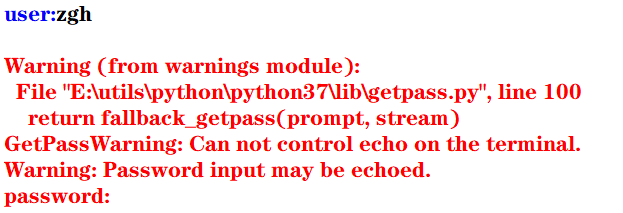user:zgh


import sys,random

n = int(sys.argv)
for i in range(n):
print(random.randrange(0,100))


import sys

total =0.0
count = 0
for line in sys.stdin:
count += 1
total += float(line)

avg = total/count
print("average:",avg)


1. 使用操作系统实用程序more逐屏显示数据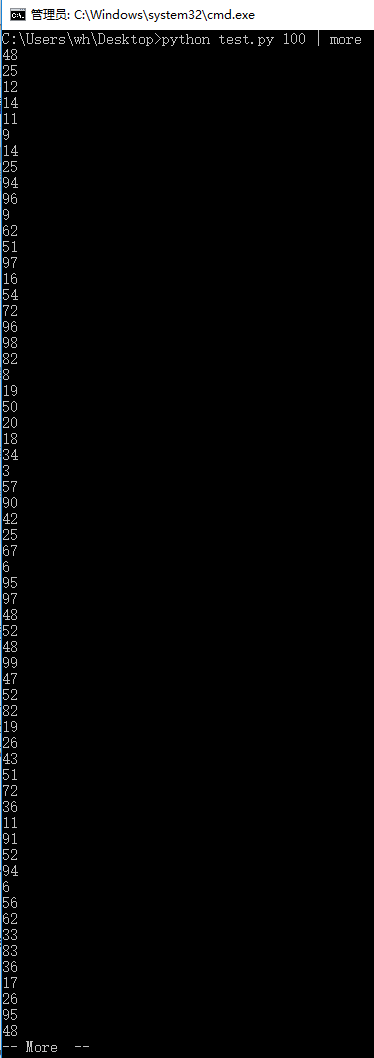2. 使用操作系统实用程序sort排序输出数据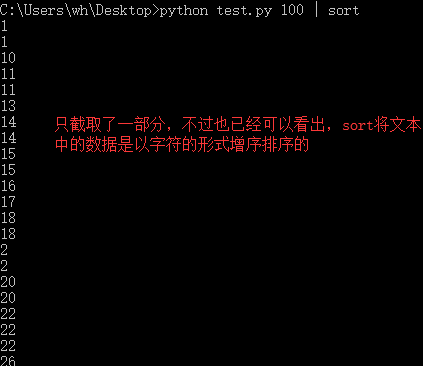more和sort都可以在一个语句中使用

## 填空题：1、2

print(value, ..., sep = ' ', end = '\n', file = sys.stdout, flush = False)

1. sep（分隔符，默认为空格）
2. end（换行符，即输入的末尾是个啥）
3. file（写入到指定文件流，默认为控制台sys.stdout）
4. flush（指定是否强制写入到流）

1

>>> print(1,2,3,4,5,sep='-',end='!')
1-2-3-4-5!


2

>>> for i in range(10):
print(i,end=' ')

0 1 2 3 4 5 6 7 8 9


## 例题及上机实践：2~5

2. 尝试修改例6.2编写的命令行参数解析的程序，解析命令行参数所输入边长的值，计算并输出正方形的周长和面积

argparse模块用于解析命名的命令行参数，生成帮助信息的Python标准模块

import argparse

parser = argparse.ArgumentParser()
parser.add_argument('--length', default = 10, type = int, help = '长度')
parser.add_argument('--width', default = 5, type = int, help = '宽度')

args = parser.parse_args()
area = args.length * args.width
print('面积 = ', area)

input()#加这一句是为了可以看到输出结果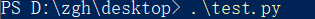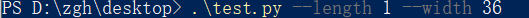import argparse

parser = argparse.ArgumentParser()
parser.add_argument('--length', default = 10, type = int, help = '长度')

args = parser.parse_args()
area = args.length ** 2
perimeter = 4 * args.length
print('面积 = {0}，周长 = {1}'.format(area,perimeter))

input()#加这一句是为了可以看到输出结果



3. 尝试修改例6.8编写读取并输出文本文件的程序，由命令行第一个参数确认所需输出的文本文件名

f = open(file, mode = 'r' , buffering = -1, encoding = None)

1. file是要打开或创建的文件名，如果文件不在当前路径，需指出具体路径
2. mode是打开文件的模式，模式有：
‘r’（只读）
‘w’（写入，写入前删除就内容）
‘x’（创建新文件，如果文件存在，则导致FileExistsError）
‘a’（追加）
‘b’（二进制文件）
‘t’（文本文件，默认值）
‘+’（更新，读写）
3. buffering表示是否使用缓存（缓存为-1，表示使用系统默认的缓冲区大小）
4. encoding是文件的编码

import sys

filename = sys.argv#就读取本文件，骚的呀皮
f = open(filename, 'r', encoding = 'utf-8')

line_no = 0
while True:
line_no += 1
if line:
print(line_no, ":", line)
else:
break
f.close()


1 : import sys

2 :

3 : filename = sys.argv#就读取本文件，骚的呀皮

4 : f = open(filename, 'r', encoding = 'utf-8')

5 :

6 : line_no = 0

7 : while True:

8 :     line_no += 1

10 :     if line:

11 :         print(line_no, ":", line)

12 :     else:

13 :         break

14 : f.close()

15 :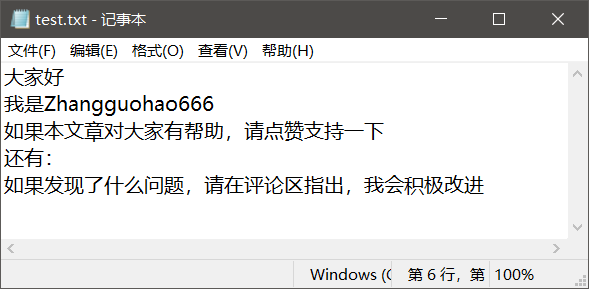1. 将test.txt文本文件的编码修改为utf-8，代码如上所说
记事本方式打开test.txt文件，点击文件，点击另存为，看到下方的编码（修改为utf-8）
2. test.txt就用默认的ANSI编码方式，再将上例代码的第三个语句修改为f = open(filename, 'r', encoding = 'ANSI')

1 : ﻿大家好

2 : 我是Zhangguohao666

3 : 如果本文章对大家有帮助，请点赞支持一下

4 : 还有：

5 : 如果发现了什么问题，请在评论区指出，我会积极改进


4. 尝试修改例6.9编写利用with语句读取并输出文本文件的程序，由命令行第一个参数确认所需输出的文本文件名

with open(file,mode) as f:

import sys

filename = sys.argv

line_no = 0
with open(filename, 'r', encoding = 'utf-8') as f:
for line in f:
line_no += 1
print(line_no, ":", line)
f.close()


import sys

filename = sys.argv

line_no = 0
with open(filename, 'r', encoding = 'ANSI') as f:
for line in f:
line_no += 1
print(line_no, ":", line)
f.close()



import sys

filename = sys.argv

line_no = 0
with open(filename, 'r', encoding = 'utf-8') as f:
for line in f:
line_no += 1
print(line_no, ":", line)
f.close()



1 : ﻿大家好

2 : 我是Zhangguohao666

3 : 如果本文章对大家有帮助，请点赞支持一下

4 : 还有：

5 : 如果发现了什么问题，请在评论区指出，我会积极改进


5. 尝试修改例6.12编写标准输出流重定向的程序，从命令行第一个参数中获取n的值，然后将0-n，0-n的2倍值，2的0-n次幂的列表打印输出到out.log文件中

1. 标准输入流文件对象：sys.stdin，
默认值为sys.__stdin__
2. 标准输出流文件对象：sys.stdout，
默认值为sys.__stdout__
3. 错误输出流文件对象（标准错误流文件对象）：sys.stderr
默认值为sys.__stderr__

import sys

n = int(sys.argv)
power = 1
i = 0

f = open('out.log', 'w')
sys.stdout = f

while i <= n:
print(str(i), ' ', str(power))
power = 2*power
i += 1
sys.stdout = sys.__stdout__


（发现文件关闭不了（就是×不掉），

（既然__stdout__不好使，就使用中间变量）

import sys

n = int(sys.argv)
power = 1
i = 0

output = sys.stdout
f = open('out.log', 'w')
sys.stdout = f

while i <= n:
print(str(i), ' ', str(power))
power = 2*power
i += 1

f.close()
sys.stdout = output
print('done!')#这一句是用来检测上面的代码是否成功执行



import sys

n = int(sys.argv)
power = 1
i = 0

output = sys.stdout
f = open('out.log', 'w')
sys.stdout = f

while i <= n:
print(str(i), ' ',  str(2*i),  ' ', str(power))
power = 2*power
i += 1

f.close()
sys.stdout = output
print('done!')#这一句是用来检测上面的代码是否成功执行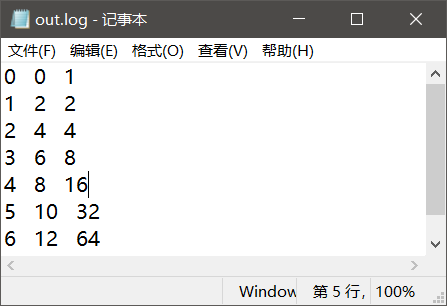## 案例研究：21点扑克牌游戏

https://blog.csdn.net/Zhangguohao666/article/details/103948545

# 第七章 错误和异常处理

Python语言采用结构化的异常处理机制捕获和处理异常

## 几个例题

1. 语法错误

1. 运行时错误

• 程序中没有导入相关的模块，NameError
• 程序中包括零除运算，ZeroDivisionError
• 程序中试图打开不存在的文件，FileNotFoundError
1. 逻辑错误

>>> a = -1
>>> if(a < 0): raise ValueError("数值不能为负数")

Traceback (most recent call last):
File "<pyshell#9>", line 1, in <module>
if(a < 0): raise ValueError("数值不能为负数")
ValueError: 数值不能为负数
>>>


try:
可能产生异常的语句
except Exception1:
发生Exception1时执行的语句
except (Exception2,Exception3):
发生Exception2或Exception3时执行的语句
except Exception4 as e:
发生Exception4时执行的语句，Exception4的实例是e
except:
捕获其他所有异常
else:
无异常时执行的语句
finally:
不管异常发生与否都保证执行的语句


except语句可以写多个，但是要注意一点：系统是自上而下匹配发生的异常，所以用户需要将带有最具体的（即派生类程度最高的）异常类的except写在前面

>>> class NumberError(Exception):
def __init__(self,data):
Exception.__init__
(self,data)
self.data = data
def __str__(self):
return self.data + '：非法数值（<0）'

>>>
>>> def total(data):
total = 0
for i in data:
if i < 0: raise NumberError(str(i))
total += 1

>>>
>>> data1 = (44, 78, 90, 80, 55)
>>> print("sum: ",total(data1))
sum:  5
>>>
>>> data2 = (44, 78, 90, 80, -1)
>>> print("sum: ",total(data2))
Traceback (most recent call last):
File "<pyshell#24>", line 1, in <module>
print("sum: ",total(data2))
File "<pyshell#18>", line 4, in total
if i < 0: raise NumberError(str(i))
NumberError: -1：非法数值（<0）
>>>


1. 用户可以使用print()函数打印输出结果
2. 也可以通过断点跟踪调试查看变量
3. 但使用断言更加灵活

assert语句和AssertionError

• assert <布尔表达式>
即：if __debug__: if not testexpression: raise AssertionError
• assert <布尔表达式>,<字符串表达式>
即：if __debug__: if not testexpression: raise AssertionError(data)
字符串表达式（即data）是断言失败时输出的失败消息

__debug__也是布尔值，Python解释器有两种：调试模式和优化模式

• 调试模式：__debug__ == True
• 优化模式：__debug__ == False

a =int(input("a: "))
b =int(input("b: "))
assert b != 0, '除数不能为零'
c = a/b
print("a/b = ", c)


cmd出场：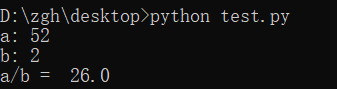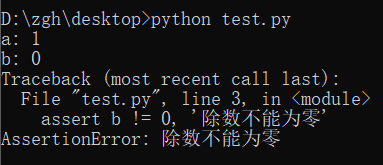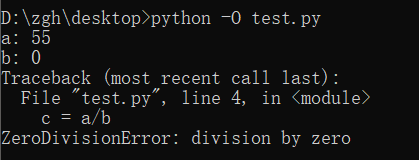## 案例研究：使用调试器调试Python程序

https://blog.csdn.net/Zhangguohao666/article/details/103948568

# 第八章 函数和函数式编程

## 一些知识点总结和几个例题

Python中函数的分类：

1. 内置函数
在程序中可以直接使用
2. 标准库函数
Python语言安装程序同时会安装若干标准库，例如math、random等
3. 第三方库函数
Python社区提供了许多其它高质量的库，在下载、安装这些库后，通过import语句可以导入库
4. 用户自定义函数
• 函数名为有效的标识符（命名规则为全小写字母，可以使用下划线增加可阅读性，例如my_func()
• 函数可以使用return返回值
如果函数体中包含return语句，则返回值
否则不返回，即返回值为空（None），无返回值的函数相当于其它编程语言中的过程

• 内置函数对象会自动创建
• import导入模块时会执行模块中的def语句，创建模块中定义的函数
• Python程序结构顺序通常为import语句>函数定义>全局代码

n = int(input("行数："))

def print_star(n):
print((" * " * n).center(50))

for i in range(1, 2*n, 2):
print_star(i)


行数：5
*
*  *  *
*  *  *  *  *
*  *  *  *  *  *  *
*  *  *  *  *  *  *  *  *


• 副作用：例如读取键盘输入，产生输出，改变系统的状态等
• 在一般情况下，产生副作用的函数相当于其它程序设计语言中的过程，可以省略return语句

def harmonic(n):
total = 0.0
for i in range(1, n+1):
total += 1.0/i

n = int(input("n："))

print("输出前n个调和数的值：")
for i in range(1, n+1):
print(harmonic(i))


 n：8

1.0
1.5
1.8333333333333333
2.083333333333333
2.283333333333333
2.4499999999999997
2.5928571428571425
2.7178571428571425


• 实际参数值默认按位置顺序依次传递给形式参数。如果参数个数不对，将会产生错误

1. 若传递的是不可变对象（例如：int、float、bool、str对象）的引用，则如果函数体中修改对象的值，其结果实际上是创建了一个新的对象
i = 1

def func(i,n):
i += n
return i

print(i)#1
func(i,10)
print(i)#1


1. 若传递的是可变对象（例如：list对象）的引用，则在函数体中可以直接修改对象的值
import random

def shuffle(a):
n = len(a)
for i in range(n):
r = random.randrange(i,n)
a[i],a[r] = a[r],a[i]

a = [1,2,3,4,5]
print("初始：",a)
shuffle(a)
print("调用函数后：",a)


初始： [1, 2, 3, 4, 5]



• 在声明函数时，如果希望函数的一些参数是可选的，可以在声明函数时为这些参数指定默认值
>>> def babbles(words, times=1):
print(words * times)

>>> babbles('Hello')
Hello
>>>
>>> babbles("Hello", 2)
HelloHello
>>>


• 位置参数：当函数调用时，实参默认按位置顺序传递形参
• 命名参数（关键字参数）：按名称指定传入的参数
参数按名称意义明确
传递的参数与顺序无关
如果有多个可选参数，则可以选择指定某个参数值

>>> def my_sum(mid_score, end_score, mid_rate = 0.4):
score = mid_score*mid_rate + end_score*(1-mid_rate)
print(format(score,'.2f'))

>>> my_sum(80,90)
86.00
>>> my_sum(mid_score = 80,end_score = 90)
86.00
>>> my_sum(end_score = 90,mid_score = 80)
86.00
>>>


• 在声明函数时，可以通过带星号的参数（例如：def func(* param)）向函数传递可变数量的实参，调用函数时，从那一点后所有的参数被收集为一个元组
• 在声明函数时，可以通过带双星号的参数（例如：def func(** param)）向函数传递可变数量的实参，调用函数时，从那一点后所有的参数被收集为一个字典

>>> def my_sum(a,b,*c):
total = a+b
for i in c:
total += i

>>> print(my_sum(1,2))
3
>>> print(my_sum(1,2,3,4,5,6))
21


>>> def my_sum(a,b,*c,**d):
total = a+b
for i in c:
total += i
for key in d:
total += d[key]

>>> print(my_sum(1,2))
3
>>> print(my_sum(1,2,3,4))
10
>>> print(my_sum(1,2,3,4,male=1,female=2))
13


• 在带星号的参数后面声明参数会导致强制命名参数（Keyword-only），然后在调用时必须显式使用命名参数传递值
• 因为按位置传递的参数默认收集为一个元组，传递给前面带星号的可变参数
>>> def my_sum(*, mid_score, end_score, mid_rate = 0.4):
score = mid_score*mid_rate + end_score*(1-mid_rate)
print(format(score,'.2f'))

>>> my_sum(mid_score=80,end_score=90)
86.00
>>> my_sum(end_score=90,mid_score=80)
86.00
>>> my_sum(80,90)
Traceback (most recent call last):
File "<pyshell#47>", line 1, in <module>
my_sum(80,90)
TypeError: my_sum() takes 0 positional arguments but 2 were given
>>>


• 在函数体中可以引用全局变量，但是要为定义在函数外的全局变量赋值，需要使用global语句
pi = 2.1415926
e = 2.7182818

def my_func():
global pi
pi = 3.14
print("global pi = ", pi)
e = 2.718
print("local e = ", e)

print('module pi = ', pi)
print('module e = ', e)
my_func()
print('module pi = ', pi)
print('module e = ', e)


module pi =  2.1415926
module e =  2.7182818
global pi =  3.14
local e =  2.718
module pi =  3.14
module e =  2.7182818

• 在函数体中可以定义嵌套函数，在嵌套函数中如果要为定义在上级函数体的局部变量赋值，可以使用nonlocal
def outer_func():
tax_rate = 0.17
print('outer function tax rate is ',tax_rate)
def inner_func():
nonlocal tax_rate
tax_rate = 0.01
print('inner function tax rate is ',tax_rate)
inner_func()
print('outer function tax rate is ',tax_rate)

outer_func()


outer function tax rate is  0.17
inner function tax rate is  0.01
outer function tax rate is  0.01

• 输出局部变量和全局变量
1. 内置函数locals()，局部变量列表
2. 内置函数globals()，全局变量列表

>>> import sys
>>> sys.getrecursionlimit()
1000
>>> sys.setrecursionlimit(666)
>>> sys.getrecursionlimit()
666
>>>


eval

• 对动态表达式进行求值，返回值
• eval(expression, globals=None, locals=None)
expression是动态表达式的字符串
globals和locals是求值时使用的上下文环境的全局变量和局部变量，如果不指定，则使用当前运行上下文
>>> x = 2
>>> str_func = input("请输入表达式：")

>>> eval(str_func)
9
>>>


exec

• 可以执行动态表达式，不返回值
• exec(str, globals=None, locals=None)
>>> exec("for i in range(10): print(i, end=' ')")
0 1 2 3 4 5 6 7 8 9
>>>


compile

• 编译代码为代码对象，可以提高效率
• compile(source, filename, mode)
source为代码语句的字符串；如果是多行语句，则每一行的结尾必须有换行符\n
filename为包含代码的文件
mode为编码方式，可以为'exec'（用于语句序列的执行），可以为'eval'（用于表达式求值），可以为'single'（用于单个交互语句）
>>> co = compile("for i in range(10): print(i, end=' ')", '', 'exec')
>>> exec(co)
0 1 2 3 4 5 6 7 8 9
>>>


• map(f, iterable,…)，将函数f应用于可迭代对象，返回结果为可迭代对象

>>> def is_odd(x):
return x%2 == 1

>>> list(map(is_odd,range(5)))
[False, True, False, True, False]
>>>


>>> list(map(abs,[1,-2,3,-4,5,-6]))
[1, 2, 3, 4, 5, 6]
>>>


>>> list(map(str,[1,2,3,4,5]))
['1', '2', '3', '4', '5']
>>


>>> def greater(x,y):
return x>y

>>> list(map(greater,[1,5,7,3,9],[2,8,4,6,0]))
[False, False, True, False, True]
>>>

• filter(f, iterable)，将函数f应用于每个元素，然后根据返回值是True还是False决定保留还是丢弃该元素，返回结果为可迭代对象

>>> def is_odd(x):
return x%2 == 1

>>> list(filter(is_odd, range(10)))
[1, 3, 5, 7, 9]
>>>


>>> list(filter(is_palindrome, range(100, 1000)))
[101, 111, 121, 131, 141, 151, 161, 171, 181, 191, 202, 212, 222, 232, 242, 252, 262, 272, 282, 292, 303, 313, 323, 333, 343, 353, 363, 373, 383, 393, 404, 414, 424, 434, 444, 454, 464, 474, 484, 494, 505, 515, 525, 535, 545, 555, 565, 575, 585, 595, 606, 616, 626, 636, 646, 656, 666, 676, 686, 696, 707, 717, 727, 737, 747, 757, 767, 777, 787, 797, 808, 818, 828, 838, 848, 858, 868, 878, 888, 898, 909, 919, 929, 939, 949, 959, 969, 979, 989, 999]
>>>


lambda arg1,arg2... : <expression>


>>> f = lambda x,y : x+y
>>> type(f)
<class 'function'>
>>> f(1,1)
2
>>>


>>> list(filter(lambda x:x%2==1, range(10)))
[1, 3, 5, 7, 9]
>>>


>>> list(filter(lambda s:s and s.strip(), ['A', '', 'B', None, 'C', ' ']))
['A', 'B', 'C']
>>>


• strip()用来去除头尾字符、空白符（\n,\r,\t,’’,即换行、回车、制表、空格）
• lstrip()用来去除开头字符、空白符
• rstrip()用来去除结尾字符、空白符

• \n到下一行的开头
• \r回到这一行的开头

>>> list(filter(lambda x:x>0, [1,0,-2,8,5]))
[1, 8, 5]
>>>


>>> list(map(lambda x:x*x, range(10)))
[0, 1, 4, 9, 16, 25, 36, 49, 64, 81]
>>>


Python内置操作符的函数接口，它定义了对应算术和比较等操作的函数，用于map()、filter()等需要传递函数对象作为参数的场合，可以直接使用而不需要使用函数定义或者Lambda表达式，使得代码更加简洁

>>> import operator
>>> a = 'hello'
>>>> operator.concat(a, ' world')
'hello world'


>>> import operator
>>> list(map(operator.gt, [1,5,7,3,9],[2,8,4,6,0]))
[False, False, True, False, True]
>>>


functools.reduce()

functools.reduce(func, iterable[, iterable[, initializer]])

• 使用指定的带两个参数的函数func对一个数据集合的所有数据进行下列操作：
• 使用第一个和第二个数据作为参数用func()函数运算，得到的结果再与第三个数据作为参数用func()函数运算，依此类推，最后得到一个结果
• 可选的initialzer为初始值

>>> import functools,operator
15
25
5050
>>>
>>> functools.reduce(operator.mul, range(1,11))
3628800


functools.partial(func, *arg, **keywords)

• 通过把一个函数的部分参数设置为默认值的方式返回一个新的可调用（callable）的partial对象
• 主要用于设置预先已知的参数，从而减少调用时传递参数的个数

>>> import functools,math
>>> pow2 = functools.partial(math.pow, 2)
>>> list(map(pow2, range(11)))
[1.0, 2.0, 4.0, 8.0, 16.0, 32.0, 64.0, 128.0, 256.0, 512.0, 1024.0]
>>>


sorted(iterable, *, key=None, reverse=False)

• iterable是待排序的可迭代对象
• key是比较函数（默认为None，按自然顺序排序）
• reverse用于指定是否逆序排序

>>> sorted([1,6,4,-2,9])
[-2, 1, 4, 6, 9]
>>> sorted([1,6,4,-2,9], reverse=True)
[9, 6, 4, 1, -2]
>>> sorted([1,6,4,-2,9], key=abs)
[1, -2, 4, 6, 9]


>>> sorted(['Dod', 'cat', 'Rabbit'])
['Dod', 'Rabbit', 'cat']
>>> sorted(['Dod', 'cat', 'Rabbit'], key=str.lower)
['cat', 'Dod', 'Rabbit']
>>> sorted(['Dod', 'cat', 'Rabbit'], key=len)
['Dod', 'cat', 'Rabbit']


>>> sorted([('Bob', 75), ('Adam', 92), ('Lisa', 88)])
[('Adam', 92), ('Bob', 75), ('Lisa', 88)]
>>> sorted([('Bob', 75), ('Adam', 92), ('Lisa', 88)], key=lambda t:t)
[('Bob', 75), ('Lisa', 88), ('Adam', 92)]


import time,functools

def timeit(func):
def wrapper(*s):
start = time.perf_counter()
func(*s)
end = time.perf_counter()
print('运行时间：', end - start)
return wrapper

@timeit
def my_sum(n):
sum = 0
for i in range(n): sum += i
print(sum)

if __name__ == '__main__':
my_sum(10_0000)


4999950000



• 首先，timeit()返回的是wrapper，而不是执行（没有小括号）
• @timeit相当于，在调用my_sum()的前一刻，会执行这么个语句：my_sum = timeit(my_sum)

def makebold(fn):
def wrapper(*s):
return "<b>" + fn(*s) + "</b>"
return wrapper

def makeitalic(fn):
def wrapper(*s):
return "<i>" + fn(*s) + "</i>"
return wrapper

@makebold
@makeitalic
def htmltags(str1):
return str1

print(htmltags('Hello'))



<b><i>Hello</i></b>


## 选择题：1~5

1

>>> print(type(lambda:None))
<class 'function'>


2

>>> f = lambda x,y:x*y
>>> f(12, 34)
408


3

>>> f1 = lambda x:x*2
>>> f2 = lambda x:x**2
>>> print(f1(f2(2)))
8


4

>>> def f1(p, **p2):
print(type(p2))

>>> f1(1, a=2)
<class 'dict'>


5

>>> def f1(a,b,c):
print(a+b)

>>> nums = (1,2,3)
>>> f1(*nums)
3


## 思考题：4~11

4

>>> d = lambda p:p*2
>>> t = lambda p:p*3
>>> x = 2
>>> x = d(x)
>>> x = t(x)
>>> x = d(x)
>>> print(x)
24


5

>>> i = map(lambda x:x**2, (1,2,3))
>>> for t in i:
print(t, end=' ')

1 4 9


6

>>> def f1():
"simple function"
pass

>>> print(f1.__doc__)
simple function


7

>>> counter = 1
>>> num = 0
>>> def TestVariable():
global counter
for i in (1, 2, 3) : counter += 1
num = 10

>>> TestVariable()
>>> print(counter, num)
4 0


8

>>> def f(a,b):
if b==0 : print(a)
else : f(b, a%b)

>>> print(f(9,6))
3
None


9

>>> def aFunction():
"The quick brown fox"
return 1

>>> print(aFunction.__doc__[4:9])
quick


10

>>> def judge(param1, *param2):
print(type(param2))
print(param2)

>>> judge(1, 2, 3, 4, 5)
<class 'tuple'>
(2, 3, 4, 5)


11

>>> def judge(param1, **param2):
print(type(param2))
print(param2)

>>> judge(1, a=2, b=3, c=4, d=5)
<class 'dict'>
{'a': 2, 'b': 3, 'c': 4, 'd': 5}


## 上机实践：2~5

2. 编写程序，定义一个求阶乘的函数fact(n)，并编写测试代码，要求输入整数n（n>=0）。请分别使用递归和非递归方式实现

def fact(n):
if n == 0 :
return 1
return n*fact(n-1)

n = int(input("请输入整数n（n>=0）："))
print(str(n)+" ! =  " + str(fact(n)))



def fact(n):
t = 1
for i in range(1,n+1):
t *= i
return t

n = int(input("请输入整数n（n>=0）："))
print(str(n)+" ! =  " + str(fact(n)))



请输入整数n（n>=0）：5
5 ! =  120


3. 编写程序，定义一个求Fibonacci数列的函数fib(n)，并编写测试代码，输出前20项（每项宽度5个字符位置，右对齐），每行输出10个。请分别使用递归和非递归方式实现

def fib(n):
if (n == 1 or n == 2):
return 1
return fib(n-1)+fib(n-2)

for i in range(1,21):
print(str(fib(i)).rjust(5,' '),end = ' ')
if i %10 == 0:
print()


def fib(n):
if (n == 1 or n == 2):
return 1
n1 = n2 = 1
for i  in range(3,n+1):
n3 = n1+n2
n1 = n2
n2 = n3
return n3

for i in range(1,21):
print(str(fib(i)).rjust(5,' '),end = ' ')
if i %10 == 0:
print()


    1     1     2     3     5     8    13    21    34    55
89   144   233   377   610   987  1597  2584  4181  6765


4. 编写程序，利用可变参数定义一个求任意个数数值的最小值的函数min_n(a,b,*c)，并编写测试代码。例如对于“print(min_n(8, 2))”以及“print(min_n(16, 1, 7, 4, 15))”的测试代码

def min_n(a,b,*c):
min_number = a if(a < b) else b
for n in c:
if n < min_number:
min_number = n
return min_number

print(min_n(8, 2))
print(min_n(16, 1, 7, 4, 15))


2
1


5. 编写程序，利用元组作为函数的返回值，求序列类型中的最大值、最小值和元素个数，并编写测试代码，假设测试代码数据分别为s1=[9, 7, 8, 3, 2, 1, 55, 6]、s2=[“apple”, “pear”, “melon”, “kiwi”]和s3="TheQuickBrownFox"

def func(n):
return (max(n),min(n),len(n))

s1=[9, 7, 8, 3, 2, 1, 55, 6]
s2=["apple", "pear", "melon", "kiwi"]
s3="TheQuickBrownFox"

for i in (s1,s2,s3):
print("list = ", i)
t = func(i)
print("最大值 = {0}，最小值 = {1}，元素个数 = {2}".format(t, t, t))


list =  [9, 7, 8, 3, 2, 1, 55, 6]

list =  ['apple', 'pear', 'melon', 'kiwi']

list =  TheQuickBrownFox



## 案例研究：井字棋游戏

https://blog.csdn.net/Zhangguohao666/article/details/103280740

# 第十三章 图形绘制

## 图形绘制模块：tkinter

2. 参考例13.2利用Canvas组件创建绘制矩形的程序，尝试改变矩形边框颜色以及填充颜色

from tkinter import *

root = Tk()
c = Canvas(root, bg = 'white', width = 130, height = 70)
c.pack()

c.create_rectangle(10, 10, 60, 60, fill = 'red')
c.create_rectangle(70, 10, 120, 60, fill = 'green', outline = 'blue', width = 5)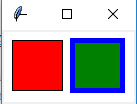• root = Tk()
创建一个Tk根窗口组件root
• c = Canvas(root, bg = 'white', width = 130, height = 70)
创建大小为200 * 100、背景颜色为白色的画布
• c.pack()
调用组件pack()方法，调整其显示位置和大小

c.create_rectangle(x0, y0, x1, y1, option, ...)

• （x0，y0）是左上角的坐标
• （x1，y1）是右下角的坐标
• c.create_rectangle(70, 10, 120, 60, fill = 'green', outline = 'blue', width = 5)
用蓝色边框、绿色填充矩形，边框宽度为5

3. 参考例13.3利用Canvas组件创建绘制椭圆的程序，尝试修改椭圆边框样式、边框颜色以及填充颜色

from tkinter import *

root = Tk()
c = Canvas(root, bg = 'white', width = 280, height = 70)
c.pack()

c.create_oval(10, 10, 60, 60, fill = 'green')
c.create_oval(70, 10, 120, 60, fill = 'green', outline = 'red', width = 5)
c.create_oval(130, 25, 180, 45, dash = (10,))
c.create_oval(190, 10, 270, 50, dash = (1,), width = 2)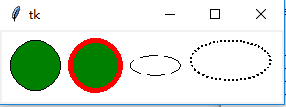c.create_oval(x0, y0, x1, y1, option, ...)

• （x0，y0）是左上角的坐标
• （x1，y1）是右下角的坐标
• c.create_oval(70, 10, 120, 60, fill = 'green', outline = 'red', width = 5)
绿色填充、红色边框，宽度为5
• c.create_oval(130, 25, 180, 45, dash = (10,))
虚线椭圆

4. 参考例13.4利用Canvas组件创建绘制圆弧的程序，尝试修改圆弧样式、边框颜色以及填充颜色

from tkinter import *

root = Tk()
c = Canvas(root, bg = 'white', width = 250, height = 70)
c.pack()

c.create_arc(10, 10, 60, 60, style = ARC)
c.create_arc(70, 10, 120, 60, style = CHORD)
c.create_arc(130, 10, 180, 60, style = PIESLICE)
for i in range(0, 360, 60):
c.create_arc(190, 10, 240, 60, fill = 'green', outline = 'red', start = i, extent = 30)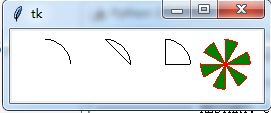c.create_arc(x0, y0, x1, y1, option, ...)

• （x0，y0）是左上角的坐标
• （x1，y1）是右下角的坐标
• 选项start（开始角度，默认为0）和extend（圆弧角度，从start开始逆时针旋转，默认为90度）决定圆弧的角度范围
• 选项start用于设置圆弧的样式

5. 参考例13.5利用Canvas组件创建绘制线条的程序，尝试修改线条样式和颜色

from tkinter import *

root = Tk()
c = Canvas(root, bg = 'white', width = 250, height = 70)
c.pack()

c.create_line(10, 10, 60, 60, arrow = BOTH, arrowshape = (3, 4, 5))
c.create_line(70, 10, 95, 10, 120, 60, fill = 'red')
c.create_line(130, 10, 180, 10, 130, 60, 180, 60, fill = 'green', width = 10, arrow = BOTH, joinstyle = MITER)
c.create_line(190, 10, 240, 10, 190, 60, 240, 60, width = 10)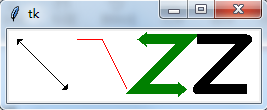c.create_line(x0, y0, x1, y1, ..., xn, yn, option, ...)

• （x0，y0）,（x1，y1），…，（xn，yn）是线条上各个点的坐标

6. 参考例13.6利用Canvas组件创建绘制多边形的程序，尝试修改多边形的形状、线条样式和填充颜色

from tkinter import *

root = Tk()
c = Canvas(root, bg = 'white', width = 250, height = 70)
c.pack()

c.create_polygon(35, 10, 10, 60, 60, 60, fill = 'red', outline = 'green')
c.create_polygon(70, 10, 120, 10, 120, 60, fill = 'white', outline = 'blue')
c.create_polygon(130, 10, 180, 10, 180, 60, 130, 60, outline = 'blue')
c.create_polygon(190, 10, 240, 10, 190, 60, 240, 60, fill = 'white', outline = 'black')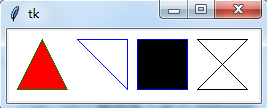c.create_polygon(x0, y0, x1, y1, ..., option, ...)

• （x0，y0）,（x1，y1），…，（xn，yn）是多边形上各个顶点的坐标

7. 参考例13.7利用Canvas组件创建绘制字符串和图形的程序，绘制y = cos(x) 的图形

c.create_text(x, y, option, ...)

• （x，y）是字符串放置的中心位置

y = sin(x)

from tkinter import *
import math

WIDTH, HEIGHT = 510, 210
ORIGIN_X, ORIGIN_Y = 2, HEIGHT/2 #原点

SCALE_X, SCALE_Y = 40, 100 #x轴、y轴缩放倍数
ox, oy = 0, 0
x, y = 0, 0
arc = 0 #弧度
END_ARC = 360 * 2 #函数图形画两个周期

root = Tk()
c = Canvas(root, bg = 'white', width = WIDTH, height = HEIGHT)
c.pack()

c.create_text(200, 20, text = 'y = sin(x)')
c.create_line(0, ORIGIN_Y, WIDTH, ORIGIN_Y)
c.create_line(ORIGIN_X, 0, ORIGIN_X, HEIGHT) #绘制x轴，y轴
for i in range(0, END_ARC+1, 10):
arc = math.pi * i / 180
x = ORIGIN_X + arc * SCALE_X
y = ORIGIN_Y - math.sin(arc) * SCALE_Y
c.create_line(ox, oy, x, y)
ox, oy = x, y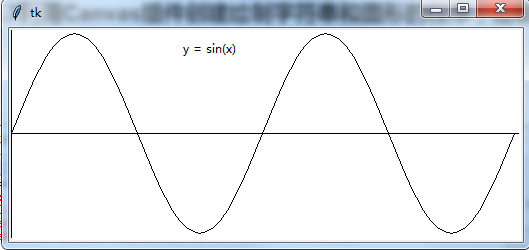y = cos(x)

from tkinter import *
import math

WIDTH, HEIGHT = 510, 210
ORIGIN_X, ORIGIN_Y = 2, HEIGHT/2 #原点

SCALE_X, SCALE_Y = 40, 100 #x轴、y轴缩放倍数
ox, oy = 0, 0
x, y = 0, 0
arc = 0 #弧度
END_ARC = 360 * 2 #函数图形画两个周期

root = Tk()
c = Canvas(root, bg = 'white', width = WIDTH, height = HEIGHT)
c.pack()

c.create_text(200, 20, text = 'y = cos(x)')
c.create_line(0, ORIGIN_Y, WIDTH, ORIGIN_Y)
c.create_line(ORIGIN_X, 0, ORIGIN_X, HEIGHT)
for i in range(0, END_ARC+1, 10):
arc = math.pi * i / 180
x = ORIGIN_X + arc * SCALE_X
y = ORIGIN_Y - math.cos(arc) * SCALE_Y
c.create_line(ox, oy, x, y)
ox, oy = x, y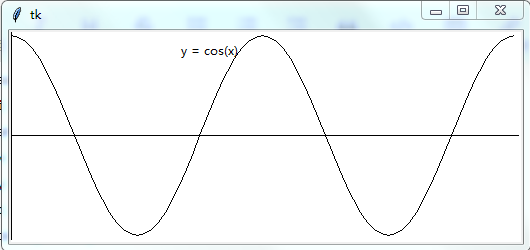# 第二十章 系统管理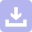下载
python核心编程第二版习题答案
10-21下载
Python程序设计算法基础教程第二版江红 青松，第十章课后习题答案
12-20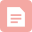博客
Python程序设计算法基础教程第二版江红 青松，第十一章课后习题答案
05-071万+博客

09-178626博客
Python程序设计算法基础教程第二版江红 青松 课后选择题 课后填空题答案
11-241万+博客
Python程序设计算法基础-----书本上机实践题目及答案

06-205万+博客
python程序设计算法基础江红答案_Python程序设计算法基础教程第二版江红 青松，第十一章课后习题答案...
12-051922博客
Python程序设计算法基础教程第二版微课第四章上机实践答案
04-164250博客
Python程序设计算法基础教程第二版微课第二上机实践答案
03-275996博客
Python程序设计基础第2by董付国 习题答案
11-123207博客
Python核心编程第13章 面向对象编程 练习
06-031892博客
python程序设计算法基础教程微课课后答案_Python程序设计算法基础教程
12-042629博客
python程序设计算法基础第二版课后答案_python算法程序设计基础答案
11-24823博客
Python程序设计算法基础教程第二版江红 青松，第九章课后习题答案
05-071万+博客
python算法程序设计基础第二版-算法程序设计基础(Python) - 吴萍
11-01548博客
Python程序设计算法基础教程(第二版)第十一章 算法数据结构基础 上机实践

02-201256博客
Python语言程序设计基础第2第二课后习题答案
02-146150博客

01-302249

### “相关推荐”对你有帮助么？

•非常没帮助
•没帮助
•一般
•有帮助
•非常有帮助点击重新获取扫码支付余额充值